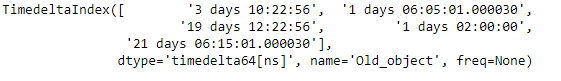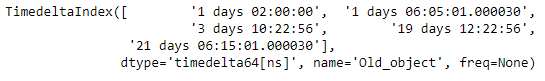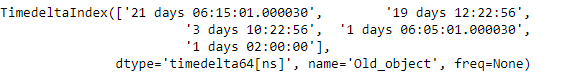Related Articles
Python | Pandas TimedeltaIndex.sort_values
• Last Updated : 06 Jan, 2019

Python is a great language for doing data analysis, primarily because of the fantastic ecosystem of data-centric python packages. Pandas is one of those packages and makes importing and analyzing data much easier.

Pandas` TimedeltaIndex.sort_values()` function return a sorted copy of the given TimedeltaIndex object.

Syntax : TimedeltaIndex.sort_values(return_indexer=False, ascending=True)

Parameters :
ascending : decides order

Return : object of same type

Example #1: Use `TimedeltaIndex.sort_values()` function to sort the given TimedeltaIndex object in ascending order.

 `# importing pandas as pd``import` `pandas as pd`` ` `# Create the TimedeltaIndex object``tidx ``=` `pd.TimedeltaIndex(data ``=``[``'3 days 10:22:56'``, ``'1 days 06:05:01.000030'``,``                                   ``'19 days 12:22:56'``, ``'1 days 02:00:00'``,``                           ``'21 days 06:15:01.000030'``], name ``=``'Old_object'``)`` ` `# Print the TimedeltaIndex object``print``(tidx)`

Output :Now we will use the `TimedeltaIndex.sort_values()` function to sort tidx object.

 `# sort the values in the given ``# object in ascending order``tidx.sort_values()`

Output :As we can see in the output, the `TimedeltaIndex.sort_values()` function has returned an object which is sorted in ascending order.

Example #2: Use `TimedeltaIndex.sort_values()` function to sort the given TimedeltaIndex object in descending order.

 `# importing pandas as pd``import` `pandas as pd`` ` `# Create the TimedeltaIndex object``tidx ``=` `pd.TimedeltaIndex(data ``=``[``'3 days 10:22:56'``, ``'1 days 06:05:01.000030'``,``                             ``'19 days 12:22:56'``, ``'1 days 02:00:00'``,``                           ``'21 days 06:15:01.000030'``], name ``=``'Old_object'``)`` ` `# Print the TimedeltaIndex object``print``(tidx)`

Output :Now we will use the `TimedeltaIndex.sort_values()` function to sort tidx object.

 `# sort the values in the given object in descending order``tidx.sort_values(ascending ``=` `False``)`

Output :As we can see in the output, the `TimedeltaIndex.sort_values()` function has returned an object which is sorted in descending order.

Attention geek! Strengthen your foundations with the Python Programming Foundation Course and learn the basics.

To begin with, your interview preparations Enhance your Data Structures concepts with the Python DS Course.

My Personal Notes arrow_drop_up